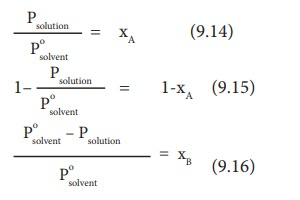Home | | Chemistry 11th std | Vapour pressure of liquid solutions

# Vapour pressure of liquid solutions

When a solute (of any physical state - solid, liquid or gas ) is dissolved in a liquid solvent the resultant solution is called a liquid solution.

Vapour pressure of liquid solutions

When a solute (of any physical state - solid, liquid or gas ) is dissolved in a liquid solvent the resultant solution is called a liquid solution. The solution which contains only two components (one solvent and one solute) is called a binary solution. We have already discussed the solution of a gaseous solute in liquid solvent under Henry's law.

## Vapour pressure of binary solution of liquid in liquids

Now, let us consider a binary liquid solution formed by dissolving a liquid solute ŌĆśAŌĆÖ in a pure solvent ŌĆśBŌĆÖ in a closed vessel. Both the components A and B present in the solution would evaporate and an equilibrium will be established between the liquid and vapour phases of the components A and B.

The French chemist Raoult, proposed a quantitative relationship between the partial pressures and the mole fractions of two components A & B, which is known as RaoultŌĆÖs Law. This law states that ŌĆ£in the case of a solution of volatile liquids, the partial vapour pressure of each component (A & B) of the solution is directly proportional to its mole fractionŌĆØ.

### According to RaoultŌĆÖs law,

pA╬▒ xA (9.3)

pA = k xA

when xA = 1, k = p┬░A

where p┬░A is the vapour pressure of pure component ŌĆśAŌĆÖ at the same temperature.

Therefore,

pA = p┬░AXA  (9.4)

Similarly, for component ŌĆśBŌĆÖ

pB= p┬░B xB    (9.5)

xA and xB are the mole fraction of the components A and B respectively.

According to DaltonŌĆÖs law of partial pressure the total pressure in a closed vessel will be equal to the sum of the partial pressures of the individual components.

Hence,

Ptotal = pA + pB (9.6)

Substituting the values of pA and pB from equations (9.4) and (9.5) in the above equation,

Ptotal = xAp┬░A + xBp┬░B (9.7)

We know that xA + xB = 1 or xA = 1 - xB

Therefore,

Ptotal = (1 - xB) p┬░A + xB p┬░B (9.8)

Ptotal= p┬░A + xB( p┬░B- p┬░A) (9.9)

The above equation is of the straight-line equation form y = mx+c. The plot of Ptotal versus xa will give a straight line with (pB┬░- pA┬░) as slope and pA┬░ as the y intercept.

Let us consider the liquid solution containing toluene (solute) in benzene (solvent).

The variation of vapour pressure of pure benzene and toluene with its mole fraction is given in the graph.The vapour pressures of pure toluene and pure benzene are 22.3 and 74.7 mmHg, respectively. The above graph shows, the partial vapour pressure of the pure components increases linearly with the increase in the mole fraction of the respective components. The total pressure at any composition of the solute and solvent is given by the following straight line (represented as red line) equation.## Vapour pressure of binary solution of solids in liquids

When a nonvolatile solute is dissolved in a pure solvent, the vapour pressure of the pure solvent will decrease. In such solutions, the vapour pressure of the solution will depend only on the solvent molecules as the solute is nonvolatile.

For example, when sodium chloride is added to the water, the vapour pressure of the salt solution is lowered. The vapour pressure of the solution is determined by the number of molecules of the solvent present in the surface at any time and is proportional to the mole fraction of the solvent.Psolution ŌłØxA (9.11)

Where xA is the mole fraction of the solvent

Psolution    =   k xA  (9.12)

When xA  = 1,    K   = Posolvent

(Psolvento is the partial pressure of pure solvent)

Psolution    =   Posolvent xA  (9.13)Where xB is the fraction of the solute

(Ōł┤ xA + xB = 1, xB = 1 - xA)

The above expression gives the relative lowering of vapour pressure. Based on this expression, RaoultŌĆÖs Law can also be stated as ŌĆ£the relative lowering of vapour pressure of an ideal solution containing the nonvolatile solute is equal to the mole fraction of the solute at a given temperatureŌĆØ.## Comparison of RaoultŌĆÖs law and HenryŌĆÖs law

According to RaoultŌĆÖs law, for a solution containing a nonvolatile solute

psolute = p┬░solutexsolute (9.17)

According to HenryŌĆÖs law:

psolute = KHxsolute in solution (9.18)

The difference between the above two expressions is the proportionality constant p┬░A (Raoults Law) and KH .(Henry's Law). Henry's law is applicable to solution containing gaseous solute in liquid solvent, while the Raoults Law is applicable to nonvolatile solid solute in a liquid solvent. If the solute is non volatile then the Henry's law constant will become equal to the vapour of the pure solvent (p┬░) and thus, RaoultŌĆÖs law becomes a special Acase of HenryŌĆÖs law. For very dilute solutions the solvent obeys RaoultŌĆÖs law and the solute obeys HenryŌĆÖs law.

Tags : Chemistry , 11th Chemistry : UNIT 9 : Solutions
Study Material, Lecturing Notes, Assignment, Reference, Wiki description explanation, brief detail
11th Chemistry : UNIT 9 : Solutions : Vapour pressure of liquid solutions | Chemistry